Next: Interval Arithmetic in LEDA Up: Number Types and Linear Previous: The data type bigfloat   Contents   Index

# The data type real ( real )

Definition

An instance x of the data type real is a real algebraic number. There are many ways to construct a real: either by conversion from double, bigfloat, integer or rational, by applying one of the arithmetic operators + , - ,*,/ or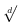to real numbers or by using the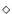-operator to define a real root of a polynomial over real numbers. One may test the sign of a real number or compare two real numbers by any of the comparison relations = ,! = , < , < = , > and > =. The outcome of such a test is mathematically exact. We give consider an example expression to clarify this: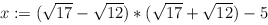Clearly, the value of x is zero. But if you evaluate x using double arithmetic you obtain a tiny non-zero value due to rounding errors. If the data type real is used to compute x then sign(x) yields zero. 1 There is also a non-standard version of the sign function: the call x.sign(integer q) computes the sign of x under the precondition that | x| < = 2-q implies x = 0. This version of the sign function allows the user to assist the data type in the computation of the sign of x, see the example below.

There are several functions to compute approximations of reals. The calls x.to_bigfloat() and x.get_bigfloat_error() return bigfloats xnum and xerr such that | xnum - x| < = xerr. The user may set a bound on xerr. More precisely, after the call x.improve_approximation_to(integer q) the data type guarantees xerr < = 2-q. One can also ask for double approximations of a real number x. The calls x.to_double() and x.get_double_error() return doubles xnum and xerr such that | xnum - x| < = xerr. Note that xerr =is possible.

#include < LEDA/numbers/real.h >

Types

 typedef polynomial Polynomial the polynomial type.

Creation

reals may be constructed from data types double, bigfloat, long, int and integer. The default constructor real() initializes the real to zero.

Operations

 double x.to_double() returns the current double approximation of x. double x.to_double(double& error) as above, but also computes a bound on the absolute error. bigfloat x.to_bigfloat() returns the current bigfloat approximation of x. double x.get_double_error() returns the absolute error of the current double approximation of x, i.e., | x - x.todouble()| < = x.getdoubleerror(). bigfloat x.get_bigfloat_error() returns the absolute error of the current bigfloat approximation of x, i.e., | x - x.tobigfloat()| < = x.getbigfloaterror(). bigfloat x.get_lower_bound() returns the lower bound of the current interval approximation of x. bigfloat x.get_upper_bound() returns the upper bound of the current interval approximation of x. rational x.high() returns a rational upper bound of the current interval approximation of x. rational x.low() returns a rational lower bound of the current interval approximation of x. double x.get_double_lower_bound() returns a double lower bound of x. double x.get_double_upper_bound() returns a double upper bound of x. bool x.possible_zero() returns true if 0 is in the current interval approximation of x integer x.separation_bound() returns the separation bound of x. integer x.sep_bfmss() returns the k-ary BFMSS separation bound of x. integer x.sep_degree_measure() returns the degree measure separation bound of x. integer x.sep_li_yap() returns the Li / Yap separation bound of x. void x.print_separation_bounds() prints the different separation bounds of x. bool x.is_general() returns true if the expression defining x contains a-operator, false otherwise. bool x.is_rational() returns true if the expression is rational, false otherwise. rational x.to_rational() returns the rational number given by the expression. Precondition is_rational() has is true. int x.compare(const real& y) returns the sign of x-y. int compare_all(const growing_array& R, int& j) compares all elements in R. It returns i such that R[i] = R[j] and i! = j. Precondition: Only two of the elements in R are equal. [ Experimental ] int x.sign() returns the sign of (the exact value of) x. int x.sign(const integer& q) as above. Precondition: The user guarantees that | x| < = 2-q is only possible if x = 0. This advanced version of the sign function should be applied only by the experienced user. It gives an improvement over the plain sign function only in some cases. void x.improve_approximation_to(const integer& q) recomputes the approximation of x  if necessary; the resulting error of the bigfloat approximation satisfies x.getbigfloaterror() < = 2-q void x.compute_with_precision(long k) recomputes the bigfloat approximation of x, if necessary; each numerical operation is carried out with a mantissa length of k. Note that here the size of the resulting x.get_bigfloat_error() cannot be predicted in general. void x.guarantee_relative_error(long k) recomputes an approximation of x, if necessary; the relative error of the resulting bigfloat approximation is less than 2-k, i.e., x.getbigfloaterror() < = | x|*2-k. ostream& ostream& O « const real& x writes the closest interval that is known to contain x to the output stream O. Note that the exact representation of x is lost in the stream output. istream& istream& I » real& x reads x number x from the output stream I in double format. Note that stream input is currently impossible for more general types of reals. real sqrt(const real& x)real root(const real& x, int d), precondition: d > = 2

Note: The functions real_roots and diamond below are all experimental if they are applied to a polynomial which is not square-free.

 int real_roots(const Polynomial& P, list& roots, algorithm_type algorithm, bool is_squarefree) returns all real roots of the polynomial P. int real_roots(const Polynomial& P, growing_array& roots, algorithm_type algorithm, bool is_squarefree) same as above. int real_roots(const int_Polynomial& iP, list& roots, algorithm_type algorithm = isolating_algorithm, bool is_squarefree = true) returns all real roots of the polynomial iP. real diamond(int j, const Polynomial& P, algorithm_type algorithm, bool is_squarefree) returns the j-th smallest real root of the polynomial P. real diamond(rational l, rational u, const Polynomial& P, algorithm_type algorithm, bool is_squarefree) returns the real root of the polynomial P which is in the isolating interval [l,u]. real diamond_short(rational l, rational u, const Polynomial& P, algorithm_type algorithm, bool is_squarefree) returns the real root of the polynomial P which is in the isolating interval [l,u]. Precondition (u - l ) < 1/4 real diamond(int j, const int_Polynomial& iP, algorithm_type algorithm = isolating_algorithm, bool is_squarefree = true) returns the j-th smallest real root of the polynomial iP. real diamond(rational l, rational u, const int_Polynomial& iP, algorithm_type algorithm = isolating_algorithm, bool is_squarefree = true) returns the real root of the polynomial iP which is in the isolating interval [l,u]. real abs(const real& x) absolute value of x real sqr(const real& x) square of x real dist(const real& x, const real& y) euclidean distance of point (x,y) to the origin real powi(const real& x, int n) xn, i.e., n.th power of x integer floor(const real& x) returns the largest integer smaller than or equal to x. integer ceil(const real& x) returns the smallest integer greater than or equal to x. rational small_rational_between(const real& x, const real& y) returns a rational number between x and y with the smallest available denominator. Note that the denominator does not need to be strictly minimal over all possible rationals. rational small_rational_near(const real& x, double eps) returns small_rational_between(x-eps, x+eps).

Implementation

A real is represented by the expression which defines it and an interval inclusion I that contains the exact value of the real. The arithmetic operators + , - ,*,take constant time. When the sign of a real number needs to be determined, the data type first computes a number q, if not already given as an argument to sign, such that |x| < = 2-q implies x = 0. The bound q is computed as described in . Using bigfloat arithmetic, the data type then computes an interval I of maximal length 2-q that contains x. If I contains zero, then x itself is equal to zero. Otherwise, the sign of any point in I is returned as the sign of x.

Two shortcuts are used to speed up the computation of the sign. Firstly, if the initial interval approximation already suffices to determine the sign, then no bigfloat approximation is computed at all. Secondly, the bigfloat approximation is first computed only with small precision. The precision is then roughly doubled until either the sign can be decided (i.e., if the current approximation interval does not contain zero) or the full precision 2-q is reached. This procedure makes the sign computation of a real number x adaptive in the sense that the running time of the sign computation depends on the complexity of x.

Example

We give two typical examples for the use of the data type real that arise in Computational geometry. We admit that a certain knowledge about Computational geometry is required for their full understanding. The examples deal with the Voronoi diagram of line segments and the intersection of line segments, respectively.

The following incircle test is used in the computation of Voronoi diagrams of line segments [17,14]. For i, 1 < = i < = 3, let li : aix + biy + ci = 0 be a line in two-dimensional space and let p = (0, 0) be the origin. In general, there are two circles passing through p and touching l1 and l2. The centers of these circles have homogeneuos coordinates (xv, yv, zv), whereandLet us concentrate on one of these (say, we take the plus sign in both cases). The test whether l3 intersects, touches or misses the circle amounts to determining the sign of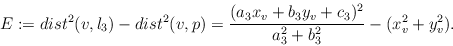The following program computes the sign of: = (a32 + b32)*E using our data type real.

int INCIRCLE( real a1, real b1, real c1, real a2, real b2, real c2, real a3, real b3, real c3 )
{
real RN = sqrt((a1*a1 + b1*b1)*(a2*a2 + b2*b2));
real RN1 = sqrt(a1*a1 + b1*b1);
real RN2 = sqrt(a2*a2 + b2*b2);
real A = a1*c2 + a2*c1;
real B = b1*c2 + b2*c1;
real C = 2*c1*c2;
real D = a1*a2 - b1*b2;
real s = b1*RN2 - b2*RN1;
real r = a1*RN2 - a2*RN1;
int signx = sign(s);
int signy = sign(r);
real xv = A + signx*sqrt(C*(RN + D));
real yv = B - signy*sqrt(C*(RN - D));
real zv = RN - (a1*a2 + b1*b2);
real P = a3*xv + b3*yv + c3*zv;
real D32 = a3*a3 + b3*b3;
real R2 = xv*xv + yv*yv;
real E = P*P - D32*R2;
return sign(E);
}

We can make the above program more efficient if all coefficients ai, bi and ci, 1 < = i < = 3, are k bit integers, i.e., integers whose absolute value is bounded by 2k - 1. In [17,14] we showed that for! = 0 we have || > = 2-24k-26. Hence we may add a parameter int k in the above program and replace the last line by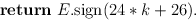Without this assistance, reals automatically compute a weaker bound of || > = 2-56k-161 for! = 0 by .

We turn to the line segment intersection problem next. Assume that all endpoints have k-bit integer homogeneous coordinates. This implies that the intersection points have homogeneous coordinates (X, Y, W) where X, Y and W are (4 k + 3) - bit integers. The Bentley-Ottmann plane sweep algorithm for segment intersection  needs to sort points by their x-coordinates, i.e., to compare fractions X1/W1 and X2/W2 where X1, X2, W1, W2 are as above. This boils down to determining the sign of the 8k + 7 bit integer X1*W2 - X2*W1. If all variables Xi, Wi are declared real then their sign test will be performed quite efficiently. First, an interval approximation is computed and then, if necessary, bigfloat approximations of increasing precision. In many cases, the interval approximation already determines the sign. In this way, the user of the data type real gets nearly the efficiency of a hand-coded floating point filter [35,66] without any work on his side. This is in marked contrast to [35,66] and will be incorporated into .Next: Interval Arithmetic in LEDA Up: Number Types and Linear Previous: The data type bigfloat   Contents   Index